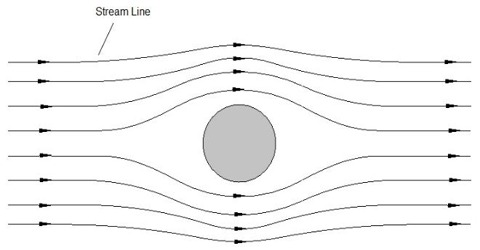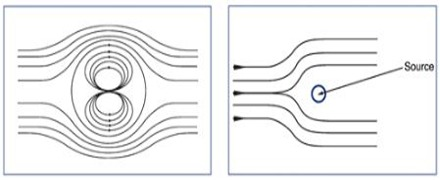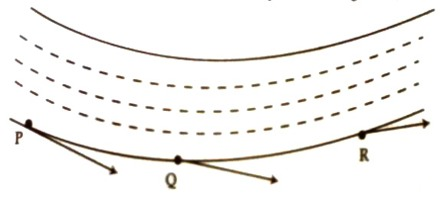# Stream-line Motion

Stream-line Motion

In streamline flow, the motion of an element following it passes an exacting point is the similar as the motion of the subdivision that preceded it at that point. The path that a element takes is called a stream line. We know, in steady flow velocity at a point remains unchanged with time. If flow of fluid is such that at any point its velocity, pressure and density remain unchanged then that flow is called stream-line flow. During streamline motion path of each particle becomes identical with paths of other particle i.e., motions of particles become parallel to each other. In fig (a) stream-line of a gas inside a cylinder has been shown and figure (b) stream-line motion of water in a pipe has been shown.In other ward, if velocity flow of each particle in a fluid at a point remains unchanged, with time, then that flow is called streamline motion. In streamline motion the path that is being followed by a tiny particle in the fluid, a tangent drawn at any point in that flow gives the direction of flow of streamline. Consider a tube of uniform cross-section and suppose that water is flowing with uniform speed through this tube. In this case flow of water is parallel to the axis. Now if speed of a particle at different points are determined, it will be seen that in every place the value is the same or in other words we can think that all particles at points P, Q, R crosses with same speed. (Fig: 2)By arrows the direction of velocity of those points has been indicated. Blood flowing in our mien is also a streamline flow.